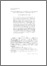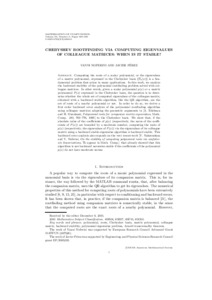# Chebyshev rootfinding via computing eigenvalues of colleague matrices: when is it stable?

Noferini, Vanni and Pérez, Javier (2016) 'Chebyshev rootfinding via computing eigenvalues of colleague matrices: when is it stable?' Mathematics of Computation, 86 (306). 1741 - 1767. ISSN 0025-5718Preview
Text
Chebyshev.pdf - Accepted Version

Download (295kB) | Preview

## Abstract

Computing the roots of a scalar polynomial, or the eigenvalues of a matrix polynomial, expressed in the Chebyshev basis {Tk(x)} is a fundamental problem that arises in many applications. In this work, we analyze the backward stability of the polynomial rootfinding problem solved with colleague matrices. In other words, given a scalar polynomial p(x) or a matrix polynomial P(x) expressed in the Chebyshev basis, the question is to determine whether or not the whole set of computed eigenvalues of the colleague matrix, obtained with a backward stable algorithm, like the QR algorithm, are the set of roots of a nearby polynomial. In order to do so, we derive a first order backward error analysis of the polynomial rootfinding algorithm using colleague matrices adapting the geometric arguments in [A. Edelman and H. Murakami, Polynomial roots for companion matrix eigenvalues, Math. Comp. 210, 763-776, 1995] to the Chebyshev basis. We show that, if the absolute value of the coefficients of p(x) (respectively, the norm of the coefficients of P(x)) are bounded by a moderate number, computing the roots of p(x) (respectively, the eigenvalues of P(x)) via the eigenvalues of its colleague matrix using a backward stable eigenvalue algorithm is backward stable. This backward error analysis also expands on the very recent work [Y. Nakatsukasa and V. Noferini, On the stability of computing polynomial roots via confederate linearizations, Math. Comp. 85 (2016), no. 301, 2391-2425] that already showed that this algorithm is not backward normwise stable if the coefficients of the polynomial p(x) do not have moderate norms.

Item Type: Article Q Science > QA Mathematics Faculty of Science and Health > Mathematical Sciences, Department of Vanni Noferini 27 Jun 2016 10:40 06 Jul 2018 13:15 http://repository.essex.ac.uk/id/eprint/17057

### Actions (login required)View Item